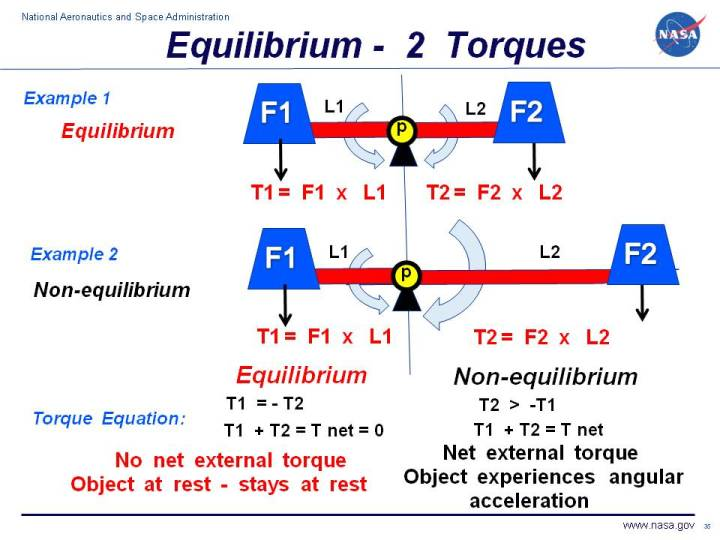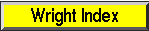+ Text Only Site
+ Non-Flash Version
+ Contact GlennA very basic concept when dealing with torques is the idea of equilibrium or balance. In general, an object can be acted on by several torques at the same time. A torque is a vector quantity which means that it has both a magnitude (size) and a direction associated with it. If the size and direction of the torques acting on an object are exactly balanced, then there is no net torque acting on the object and the object is said to be in equilibrium. Because there is no net torque acting on an object in equilibrium, an object at rest will stay at rest, and an object in constant angular motion will stay in angular motion. Let us start with the simplest example of two torques acting on an object. In Example 1 on the slide, we show a red bar, or arm, on which two blue weights are placed at either end. There is a pivot point, labeled p which is located in the exact center of the bar, breaking the bar into two equal lengths L1 and L2. The weights F1 and F2 are also of equal size. The weight F1 and arm L1 generate a torque T1 about the pivot point in the counter-clockwise direction. Similarly, the weight F2 and arm L2 generate a torque T2, but this torque is in the clockwise direction. Remember that torques are vector quantities and direction is important. Two torques with the same magnitude but different directions are not equal torques. In fact, T1 = - T2 If we sum up the torqes acting on the pivot p, we obtain the torque equation on the left: T1 + T2 = T net = 0 where T net is the net torque acting on the pivot. Because the net torque is equal to zero, the torques in Example 1 are balanced and acting in equilibrium. There is no net torque acting on the pivot in Example 1. Since the system is initially at rest (angular velocity equals zero), the system will remain at rest. If the system was rotating with a uniform angular velocity, it would continue rotating at the same angular velocity. In Example 2, we have increased the length of the arm L2 so that it is much greater than arm L1. The torque T2 is then larger than the torque T1. The torques are no longer in equilibrium. The torque equation remains the same, but the net torque is not equal to zero. The magnitude of the net torque is given by: |T net| = |T1| - |T2| where the "| |" symbols indicate the magnitude of the quantity included between the ends. The direction of the net torque would be in the clockwise direction because T1 is less then T2. If the system were initially at rest, the system would begin to accelerate clockwise. Because there is a net torque in Example 2, the torques are not in equilibrium. Because the system is pinned at point p, the system will rotate clockwise until the long arm is located directly beneath the pivot and the short arm is directly above the pivot. If angular momentum carries the system past this point, then the sense of the rotation will change and the system will rotate counter-clockwise. If there is some friction in the pivot, the system will eventually come to rest with the long arm L2 directly below the pivot. At that time, there is no net torque about the pivot and the system will again be in equilibrium. Navigation..Beginner's Guide Home Page+ Inspector General Hotline + Equal Employment Opportunity Data Posted Pursuant to the No Fear Act + Budgets, Strategic Plans and Accountability Reports + Freedom of Information Act + The President's Management Agenda + NASA Privacy Statement, Disclaimer, and Accessibility CertificationEditor: Nancy Hall NASA Official: Nancy Hall Last Updated: May 05 2015 + Contact Glenn